# Change Nickname

Current Nickname:

v4.2
Search
中文EN
v4.2

# Backpropagation

## What is Backpropagation

Backpropagation (or BP) is an abbreviation for Error Backward Propagation. BP algorithm is mainly composed of forward propagation and backpropagation processes:

• Forward propagation: Input information to the input layer of the neural network, it passes through one or more hidden layers and then outputs from the output layer.
• Backpropagation: Compare the output value with the actual value and pass the error from the output layer to the input layer via the hidden layers; in this process, adjust the weights of neurons using gradient descent technique.

The repetitive adjustments of weights is the training process of the neural network.

## Construct Neural Network

### Neural Network Structure

Neural network is normally constructed with an input layer, one or more hidden layers, and an output layer. We propose the simple neural network below as an example, sigmoid activation function is applied in the output layer: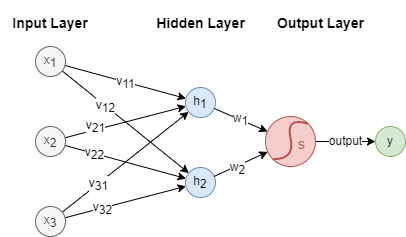### Activation Function

Activation functions empowers network to conduct non-linear modeling. Without activation functions, the network can only express linear mappings. There are a variety of activation functions, the formula and graph of the sigmoid function used here are as below: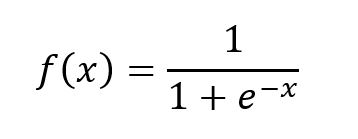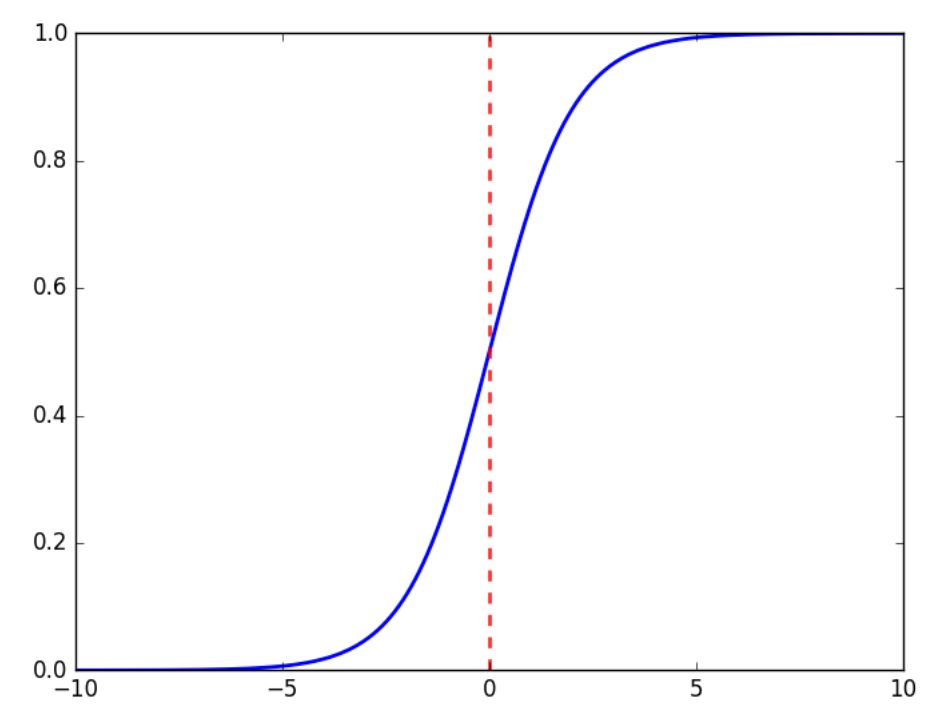### Initial Weights

Initial weights are randomly generated when the algorithm begins, we assume the initial weights are: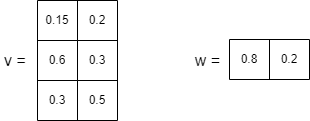### Training Samples

Suppose we have 3 groups of samples as below, the superscript represents the order of the sample:

• Inputs: x(1) = (2,3,1)，x(2) = (1,0,2)，x(3) = (3,1,1)
• Outputs: t(1) = 0.64，t(2) = 0.52，t(3) = 0.36

The goal of training is to make the output of the model (y) as close as possible to the actual output (t) when the input (x) is given.

## Forward Propagation

### Input Layer → Hidden Layer

Neurons h1 and h2 are calculated by: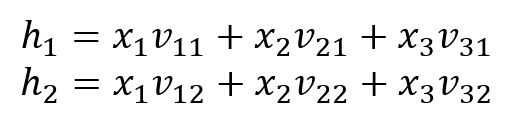### Hidden Layer → Output Layer

Output value y is calculated by: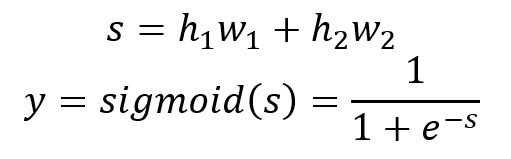### Calculation

Below is the calculation of the 3 samples:

x
h1 h2 s y t
x(1) = (2,3,1) 2.4 1.8 2.28 0.907 0.64
x(2) = (1,0,2) 0.75 1.2 0.84 0.698 0.52
x(3) = (3,1,1) 1.35 1.4 1.36 0.796 0.36

The actual output values are also listed in the table. Notice that the outputs of the 3 samples are greatly different from the expected values.

## Loss Function

Loss function is used to calculate the error between the output of the model and the expected output. Loss function is also known as objective function or cost function. A commonly used loss function is Mean-Square Error (MSE):where m is the number of samples. The error of this forward propagation is:

E = [(0.64-0.907)2 + (0.52-0.698)2 + (0.36-0.796)2] / (2*3) = 0.234

Loss function measures the accuracy of the model, the smaller the loss function value, the higher the model accuracy, and the purpose of model training is to reduce the loss function value as much as possible. Think of the input and output as constants, and the loss function as a function with weights as variables. A good way to find the weights that minimize the value of loss function is gradient descent.

## Backpropagation

Batch gradient descent (BGD) will be adopted to update weights, i.e., all samples will be involved in the calculation. The learning rate is set to η = 0.5.

### Output Layer → Hidden Layer

There are two weights w1 and w2 between output layer and hidden layer, we will adjust both respectively.

When adjusting w1, we need to see how much influence w1 has on the error E, so that to calculate partial derivative for w1 by using the chain rule：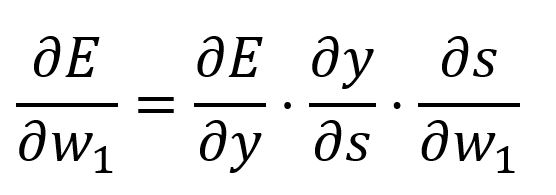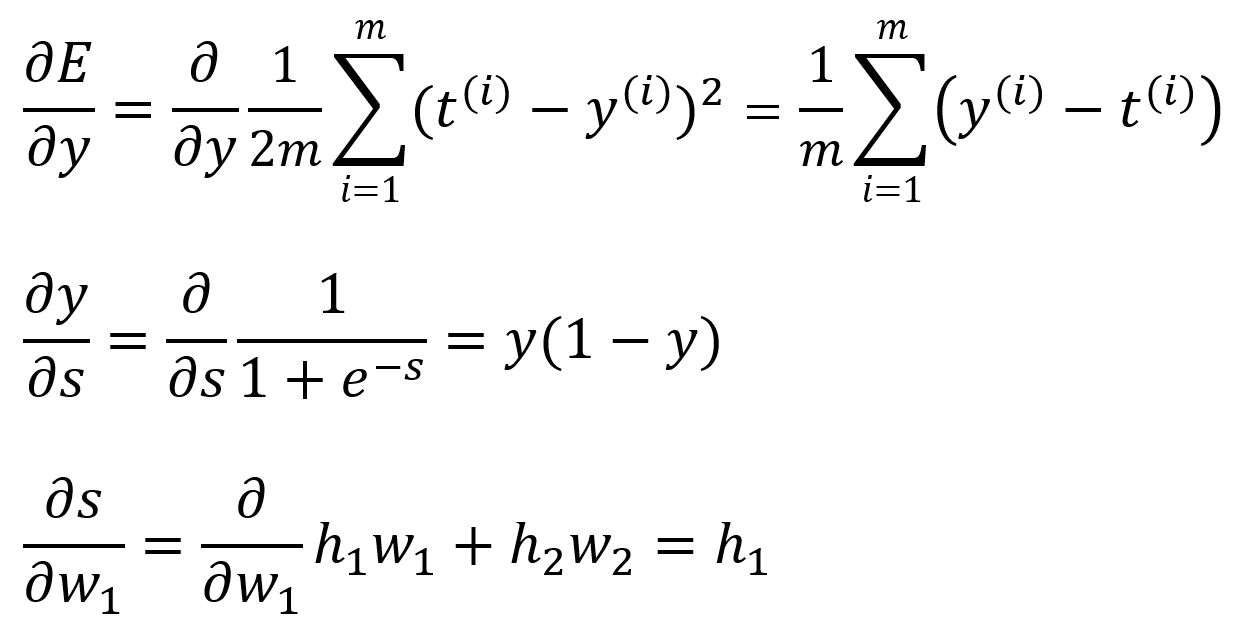Calculate with values:

∂E/∂y = [(0.907-0.64) + (0.698-0.52) + (0.796-0.36)] / 3 = 0.294
∂y/∂s = [0.907*(1-0.907) + 0.698*(1-0.698) + 0.796*(1-0.796)] / 3 = 0.152
∂s/∂w1 = (2.4 + 0.75 + 1.35) / 3 = 1.5

The final result is: ∂E/∂w1 = 0.294*0.152*1.5 = 0.067

As all 3 samples are to participate in the calculation, when calculating ∂y/∂s and ∂s/∂w1, we would need to obtain the sum and the average of them.

New w1 is w1 := w1 - η ⋅ ∂E/∂w1 = 0.8 - 0.5*0.067 = 0.766

The method to adjust w2 is similar to w1, we give the result directly here, w2 is adjusted from 0.2 to 0.167.

### Hidden Layer → Input Layer

There are 6 weights v11, v12, v21, v22, v31, and v32 between hidden layer and input layer, we will adjust each of them respectively.

When adjusting v11, we need to calculate how much influence v11 has on the error E, so that to calculate the partial derivative for v11: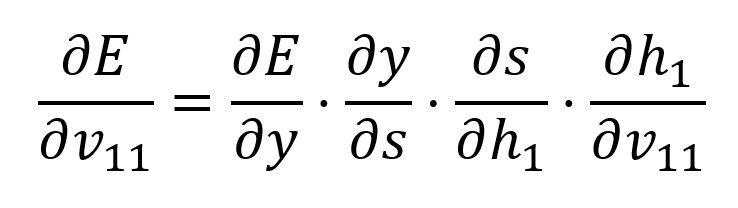We already obtained the first two gradients when adjusting w1 and w2, only need to calculate the latter two: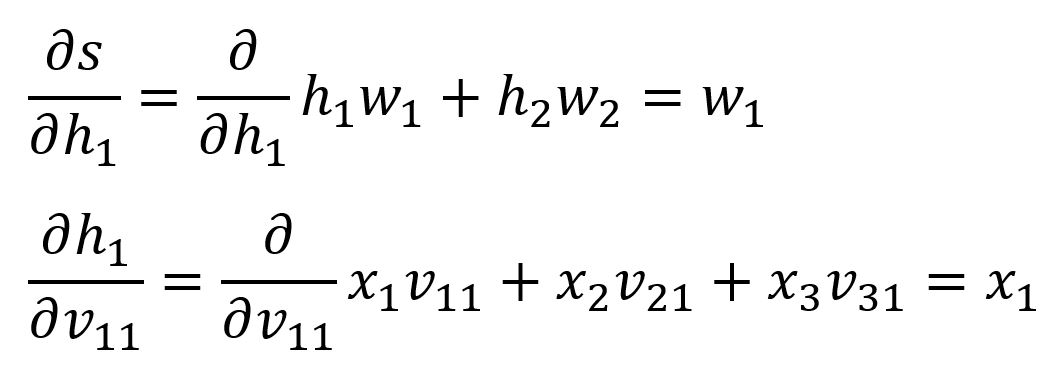Calculate with values:

∂E/∂y = 0.294
∂y/∂s = 0.152
∂s/∂h1 = 0.8
∂h1/∂v11 = (2 + 1 + 3) / 3 = 2

The final result is: ∂E/∂v11 = 0.294*0.152*0.8*2 = 0.072

New v11 is v11 := v11 - η ⋅ ∂E/∂v11 = 0.15 - 0.5*0.072 = 0.114

Adjustment for the other 5 weights is similar to v11, the results are:

• v12 is adjusted from 0.2 to 0.191
• v21 is adjusted from 0.6 to 0.576
• v22 is adjusted from 0.3 to 0.294
• v31 is adjusted from 0.3 to 0.282
• v32 is adjusted from 0.5 to 0.496

## Model Training

Apply the adjusted weights to model and use the 3 same samples to conduct forward propagation again, this time the error E = 0.192, which is obviously improved if compares with the first forward propagation with error E = 0.192.

BP algorithm repeats the forward and back-propagations to train the model iteratively until the preset training number or time is met, or the error descends to a set threshold.